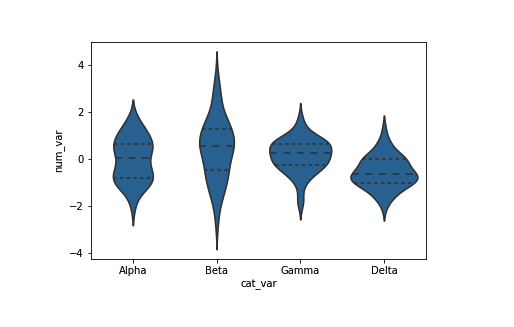Python：matplotlib 和 Seaborn 之热图、小提琴图和箱线图 (三十四)

热图

plt.figure(figsize = [12, 5])

# left plot: scatterplot of discrete data with jitter and transparency
plt.subplot(1, 2, 1)
sb.regplot(data = df, x = 'disc_var1', y = 'disc_var2', fit_reg = False,
x_jitter = 0.2, y_jitter = 0.2, scatter_kws = {'alpha' : 1/3})

# right plot: heat map with bin edges between values
plt.subplot(1, 2, 2)
bins_x = np.arange(0.5, 10.5+1, 1)
bins_y = np.arange(-0.5, 10.5+1, 1)
plt.hist2d(data = df, x = 'disc_var1', y = 'disc_var2',
bins = [bins_x, bins_y])
plt.colorbar();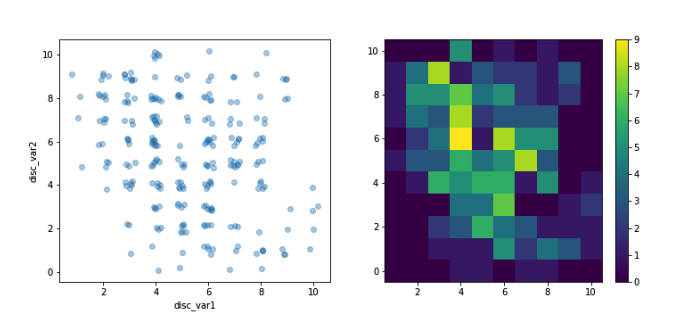其他版本

bins_x = np.arange(0.5, 10.5+1, 1)
bins_y = np.arange(-0.5, 10.5+1, 1)
plt.hist2d(data = df, x = 'disc_var1', y = 'disc_var2',
bins = [bins_x, bins_y], cmap = 'viridis_r', cmin = 0.5)
plt.colorbar()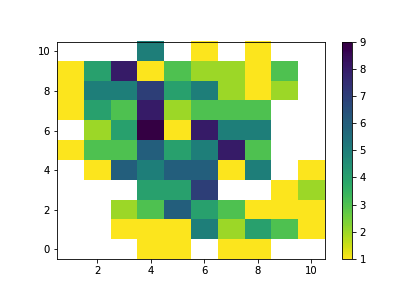# hist2d returns a number of different variables, including an array of counts
bins_x = np.arange(0.5, 10.5+1, 1)
bins_y = np.arange(-0.5, 10.5+1, 1)
h2d = plt.hist2d(data = df, x = 'disc_var1', y = 'disc_var2',
bins = [bins_x, bins_y], cmap = 'viridis_r', cmin = 0.5)
counts = h2d

# loop through the cell counts and add text annotations for each
for i in range(counts.shape):
for j in range(counts.shape):
c = counts[i,j]
if c >= 7: # increase visibility on darkest cells
plt.text(bins_x[i]+0.5, bins_y[j]+0.5, int(c),
ha = 'center', va = 'center', color = 'white')
elif c > 0:
plt.text(bins_x[i]+0.5, bins_y[j]+0.5, int(c),
ha = 'center', va = 'center', color = 'black')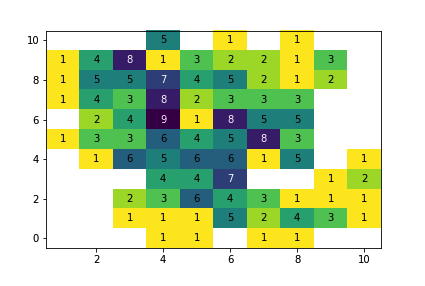小提琴图

Seaborn 的 violinplot 函数可以用来创建将小提琴图和箱线图相结合的图形，我们将在下个页面讨论箱线图。

sb.violinplot(data = df, x = 'cat_var', y = 'num_var')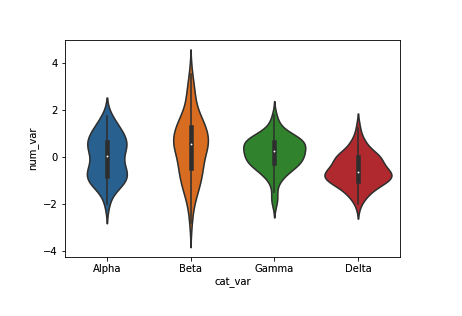base_color = sb.color_palette()
sb.violinplot(data = df, x = 'cat_var', y = 'num_var', color = base_color,
inner = None)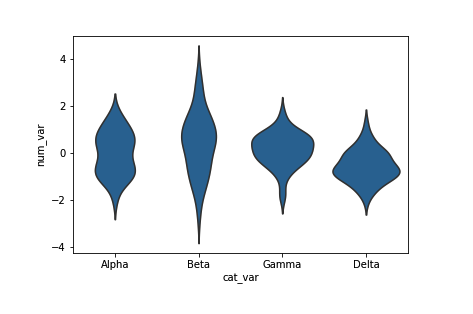其他版本

base_color = sb.color_palette()
sb.violinplot(data = df, x = 'num_var', y = 'cat_var', color = base_color,
inner = None)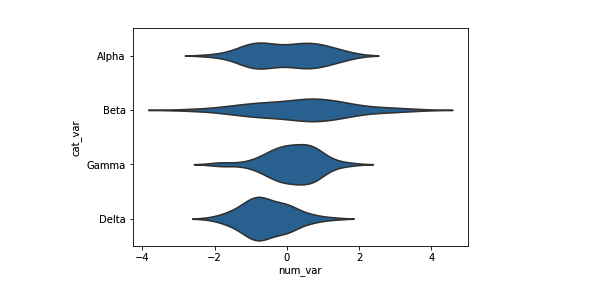箱线图

plt.figure(figsize = [10, 5])
base_color = sb.color_palette()

# left plot: violin plot
plt.subplot(1, 2, 1)
ax1 = sb.violinplot(data = df, x = 'cat_var', y = 'num_var', color = base_color)

# right plot: box plot
plt.subplot(1, 2, 2)
sb.boxplot(data = df, x = 'cat_var', y = 'num_var', color = base_color)
plt.ylim(ax1.get_ylim()) # set y-axis limits to be same as left plot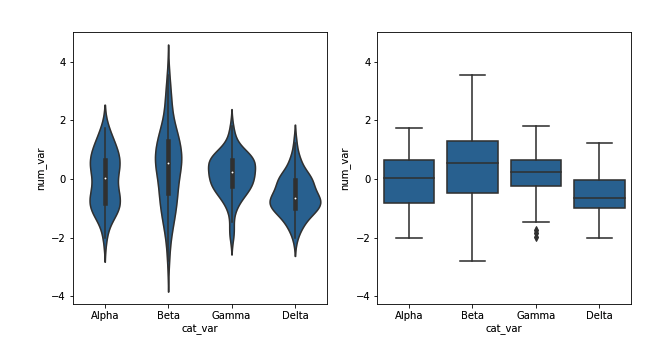其他版本

base_color = sb.color_palette()
sb.boxplot(data = df, x = 'num_var', y = 'cat_var', color = base_color)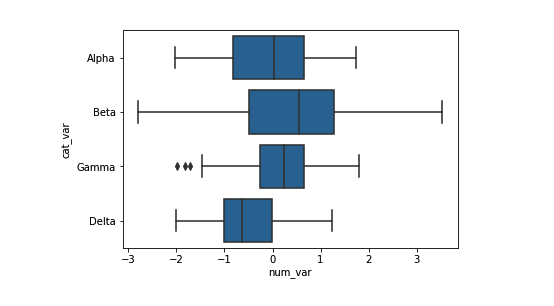base_color = sb.color_palette()
sb.violinplot(data = df, x = 'cat_var', y = 'num_var', color = base_color,
inner = 'quartile')